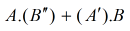Digital Electronics homework Help at TutorEye

# Best Homework Help For Digital Electronics

## Digital Electronics:

Digital electronics is a stream of electronics. It involves the study of devices related to digital signals. The electronic circuits study helps in various integrated circuits of digital electronic devices.

## Digital Electronics Sample Questions:

Question 1: The OR operation in boolean algebra is performed by which properties?

a) Commutative property     b) Associative property
c) Distributive property        d) All of these

Explanation: OR operation follows associative, commutative and distributive property. Therefore, OR operation is performed by all the above properties.

Question 2: _____________ is a process where letters or words are represented by the group of symbols.

a) Encoding                b) Decoding
c) Both (a) and (b)      d) None of these

Explanation: In order to secure the transmission of information, letters and words are denoted by different symbols. Encoding extracts codes from the computer to process the information.

Question 3: Which of the following represents absorption law?

a) A + BA = B             b) A + AB = A
c) AB + A = A             d) A + AA = A

Answer: A + BA = B

Explanation: The expression for absorption law is given by
A + AB = A(1+B) = A
A + AB = A (Since 1 + B = 1 as per 1’s property)

Question 4: Which of the following combinations is possible to construct exclusive-OR (XOR) logic gates?

a) OR                                     b) AND, NOT
c) AND, OR, NOT                  d) OR, NOT

Explanation: The expression for XOR :OR, AND, NOT are used to construct the above expression.
In total, 2 AND gates with two inputs and 1 OR are combined to construct XOR logic gates.

Question 5: How many truth tables are necessary for a three-input circuit?

a) 4                  b) 8
c) 16                d) 25

Explanation: For 3 input : 23=8
8 truth tables are necessary for a three-input circuit.

Question 6: All logic operations can be obtained by means of ____________.

a) OR and NOR                  b) OR and NOT
c) NAND and NOR             d) All of the above

Explanation: NOR and NAND are universal logic gates. They can be used to design all the three basic gates AND, OR and NOT. All the operations are obtained from these gates.

Question 7: Which of the following devices is a two stable device?

a) Latch                             b) Multivibrator
c) Monostable vibrator      d) None of these

Explanation: Latch works on the principle of bistable multivibrators. This circuit is stable in either of two states. It changes from one state to another.

Question 8: ________ is used to facilitate error correction in digital communications.

a) Gray code               b) Binary code
c) BCD code               d) ASCII code

Explanation: In digital communication systems such as television and cable system, gray codes are used to facilitate error correction.

Question 9: There are __________ types of counters?

a) 2                   b) 3
c) 4                   d) 5

Explanation: There are three counters types : asynchronous/synchronous, single and multi-mode, modulus counters.

Question 10: Which of the following counters is also called an asynchronous counter?

a) Ripple counter                 b) SSI counter
c) Decade counter                d) None of these

Explanation: In an asynchronous counter, flip flop is connected to an external clock while the rest of the flip flops have their preceding flip flop output as clock to them.